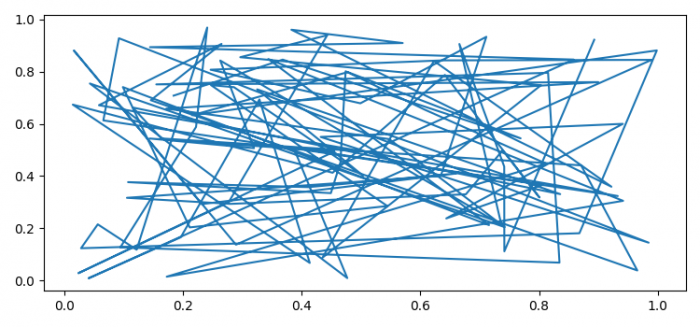# How to show Matplotlib in Flask?

To show a plot in Flask, we can take the following steps−

• Make a small application.
• To run Flask application, go to the current directory.
• $export FLASK_APP=file.py •$ flask run
• Open the browser, hit url:http://127.0.0.1:5000/print-plot/
• To plot the figure, we can create data points for x and y using random.
• Plot data points, x and y, on the created axis.
• Write a figure into png figure format.
• Retrieve the entire contents of the BytesIO object.

## Example

import io
from matplotlib.backends.backend_agg import FigureCanvasAgg as FigureCanvas
from matplotlib.figure import Figure
import numpy as np
plt.rcParams["figure.figsize"] = [7.50, 3.50]
plt.rcParams["figure.autolayout"] = True
@app.route('/print-plot')
def plot_png():
fig = Figure()
return Response(output.getvalue(), mimetype='image/png')